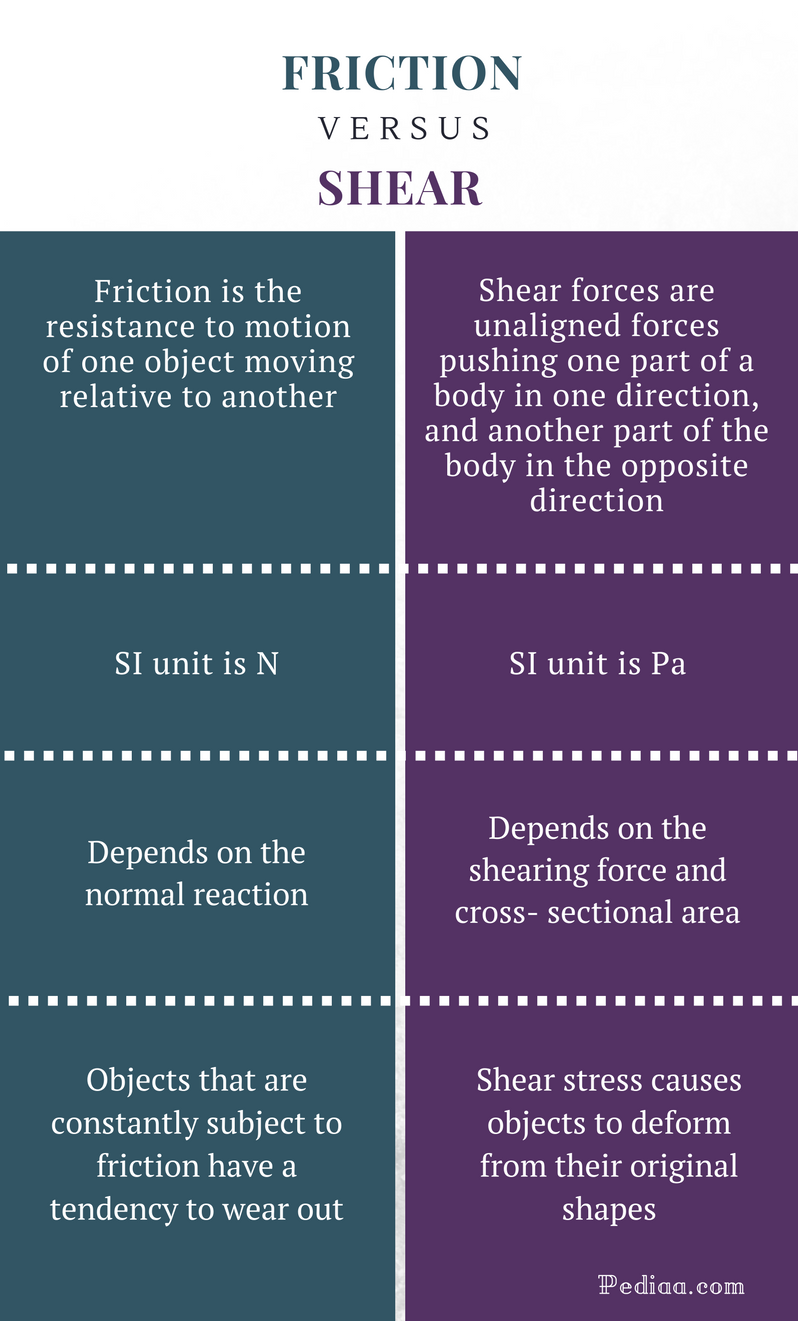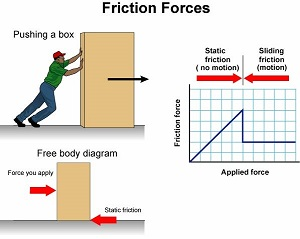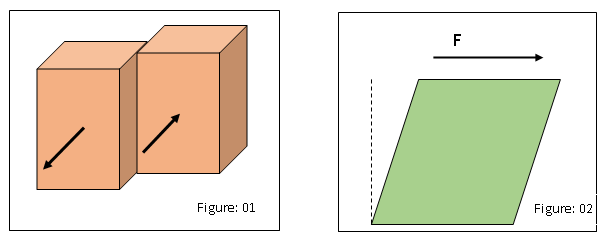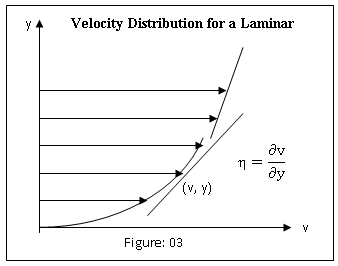# Difference Between Friction and Shear

## Main Difference – Friction vs Shear

Friction and shear stress are two phenomena which are studied especially in automobile engineering, mechanical engineering, civil engineering, and fluid dynamics. Friction is a force that opposes the relative motion of two objects (or the tendency to move) which are in contact with each other. In contrast, shear stress is a stress induced by a force. This is the main difference between friction and shear stress.

1. What is Friction? – Definition, Calculation, Features,  and Properties

2. What is Shear Stress? Definition, Calculation, Features, and Properties

3. What is the difference between Friction and Shear?## What is Friction

Friction is one of the most common types of force we experience in our daily life.  You can’t walk on a frictionless surface. You can’t stop your car if friction doesn’t exist between the tires and the road. We would have to struggle with many other critical challenges if friction didn’t exist. For example, meteors that enter the atmosphere normally burn up due to the friction between the air and meteors. But meteors would directly hit the Earth if friction doesn’t exist between air and the meteors. A world without friction is not a livable place.

When two bodies come in contact with each other, they have a tendency to move relative to each other; the forces that act between the two surfaces oppose this tendency to move. If two bodies are moving relative to each other, the forces that act between the surfaces in contact, oppose the relative motion of two bodies. These forces that oppose the tendency to move or the relative motion are known as frictional forces. Frictional forces always act in the direction opposite to the motion (or opposite to the direction of the tendency to move).

The frictional forces act tangentially to the surfaces whereas the normal reactions act perpendicular to the surfaces. In other words, normal reaction and frictional force occur perpendicular to each other. The magnitude of the frictional forces (F) between two surfaces is directly proportional to the normal reaction. It can be mathematically expressed as F = μR where R is the magnitude of normal reaction.

Frictional forces don’t only act between solid surfaces, they also between solid- liquid, solid-air, liquid-liquid layers, liquid-air and air.

There are three states of frictional forces namely; static, limiting and dynamic states. The static frictional force is the force that acts when two bodies aren’t in a motion relative to each other. The frictional force that acts when an object just begins to move relative to the other is known as the limiting frictional force. The frictional force that acts on a body which is moving relative to the other is referred to as the dynamic frictional force. The magnitude of limiting frictional force is the maximum value of the magnitude of the frictional force that could develop between two bodies. Thus, the dynamic frictional force is slightly less than the limiting frictional force.

In applications, moving parts of mechanical instruments and other equipment tend to wear out due to friction. Therefore, various methods are used to reduce the friction, especially in automobile engineering.## What is Shear

A stress arises when a shearing force is applied to an object or liquid. For example, consider two boxes which are in contact with each other. If you push one of two boxes while the other box is being pulled (as shown in figure 01), shearing forces will act along the contact surfaces of the each box. As a result, each contacting surface would experience a shear which would be induced by the shearing force. The component of the shear tangential to the surface is known as the shear stress whereas the normal component is known as the normal stress. Shear stress can be defined as the shearing force applied, divided by the area of cross section. It can be mathematically expressed as

τ = F/A

F- Shearing force applied on the object

A- Cross sectional area of the object (liquid) parallel to the force appliedShear strength is the maximum shear stress that a material can withstand without failure. Therefore, shear stress is an important factor in mechanical and civil engineering.

In fluid dynamics, shear stress is one of the frequently used technical terms. The nature of a given fluid determines how the shear stress affects that fluid. In Newtonian fluids, the shear stress is directly proportional to the strain rate, if it is a laminar flow. Therefore, for a Newtonian fluid, the shear stress (τ) can be expressed as

τ=η (∂v/∂y)

Where;

v- Velocity of the fluid at a height ‘y’ from the boundary

y- Height from the boundary

η- Viscosity of the fluid (proportionality constant)## Difference Between Friction and Shear

### Definition

Friction: Friction is the resistance to motion of one object moving relative to another.

Shear: Shear forces are unaligned forces pushing one part of a body in one direction, and another part of the body in the opposite direction.

Friction: F

Shear: τ

### Formula

Friction: F = μR

Shear: τ=η (∂v/∂y)

Friction: N

Shear: Pa (Nm-2)

### Influencing Factors

Friction: Friction depends on the normal reaction.

Shear: Shear depends on the shearing force and cross- sectional area.

### Impact

Friction: Objects that are constantly subject to friction have a tendency to wear out.

Shear: Shear stress causes an object to deform from its original shape.

Image Courtesy:

“Friction forces” By Vishakha.malhan – Own work (CC BY-SA 4.0) via Commons Wikimedia